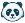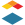I am an instructor that teaches math problem-solving courses where raw exam scores are naturally low (e.g. a 50% might be a "C" performance and 75% might be an "A"). There is a common grade-curving policy of rescaling the grades linearly, so if a student's raw score is n, then their scaled score is f(n)=an+b, where a and b are chosen parameters. In other words, the raw score is multiplied by one number, then multiplied by another.

I use this to map scores on challenging assignments into a range of numbers that is appropriate for the percentage-based letter grade system. For example, if raw scores range from 20/40 (50%) to 36/40 (90%), but the 20/40 is really a "C-" performance not an "F", I would choose a and b to map the raw scores to the range [72%, 100%].

The existing bell-curve feature does not suffice for this. The bell curve puts students in competition with one another, and I cannot control how the minimum/maximum score map to percentages.

Tags (4)Community Team
Status changed to: OpenCommunity Novice

I (an instructor) have a different curve for my frequent quizzes than I do for the rest of the class. Canvas has custom grading schemes but is not enabled for using those custom grading schemes on assignment groups (like my Quizzes assignment group) or on specific quizzes.

In a similar vein, I would also like to do a customized curve. When I curve my students' quiz grades, I don't simply raise the class average. I have a broader range for A and B grades than for C and lower grades than my normal grading scheme follows. It would be great if I could create a customized curve, e.g., converting a 50/100 to a 70/100 and converting an 80/100 to a 92/100, etc.Community Member